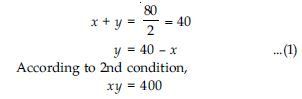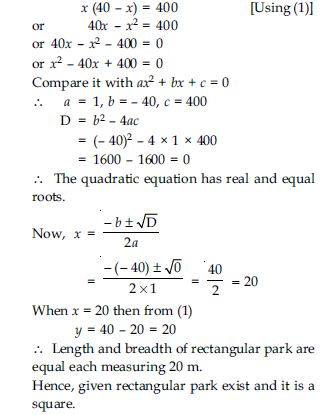# NCERT Solutions for Class 10 Math Chapter 4 - Quadratic Equations

The NCERT Solutions at Aasoka consists of questions and their solutions as per the latest NCERT syllabus. Each question is framed by experts who have years of experience in the same field. Students must practice regularly in order to score good grades in their board exams. Math is one such subject that demands regular practice. So, get started with the preparation with top NCERT Solutions for Class 10th available for free on the Aasoka platform.

Chapter 4 of Math Class 10th is “Quadratic Equations” wherein the students will learn what is a quadratic equation and the various methods to solve them. The chapter describes the square method and factorization method of solving quadratic equations. Students will get to understand the relationship between the nature of roots and discriminants. The chapter has used real-life examples to explain the equation better.

##### Question 1:

Check whether the following are quadratic equations:
(x + 1)2 = 2 (x - 3)

Given,
(x + 1)2 = 2 (x - 3)
or x2 + 1 + 2x = 2x - 6
or x2 + 1 + 2x - 2x + 6 = 0
or x2 + 7 = 0
or x2 + 0x + 7 = 0
Which is in the form of ax2 + bx + c = 0; a $\ne$ 0.
∴ It is a quadratic equation.

##### Question 2:

Check whether the following are quadratic equations:
x2 - 2x = (- 2) (3 - x)

Given,
x2 - 2x = (- 2) (3 - x)
or x2 - 2x = - 6 + 2x
or x2 - 2x + 6 - 2x = 0
or x2 - 4x + 6 = 0
Which is in the form of ax2 + bx + c = 0; a $\ne$ 0.
∴ It is a quadratic equation.

##### Question 3:

Check whether the following are quadratic equations:
(x - 2) (x + 1) = (x - 1) (x + 3)

Given,
(x - 2) (x + 1) = (x - 1) (x + 3)
or x2 + x - 2x - 2 = x2 + 3x - x - 3
or x2 - x - 2 = x2 + 2x - 3
or x2 - x - 2 - x2 - 2x + 3 = 0
or - 3x + 1 = 0
Which has no term of x2 .
So, it is not a quadratic equation.

##### Question 4:

Check whether the following are quadratic equations:
(x - 3) (2x + 1) = x (x + 5)

Given,
(x - 3) (2x + 1) = x (x + 5)
or 2x2 + x - 6x - 3 = x2 + 5x
or 2x2 - 5x - 3 - x2 - 5x = 0
or x2 - 10x - 3 = 0
Which is in the form of ax2 + bx + c = 0; a $\ne$ 0.
∴ It is a quadratic equation.

##### Question 5:

Check whether the following are quadratic equations:
(2x - 1) (x - 3) = (x + 5) (x - 1)

Given,
(2x - 1) (x - 3) = (x + 5) (x - 1)
or 2x2 - 6x - x + 3 = x2 - x + 5x - 5
or 2x2 - 7x + 3 = x2 + 4x - 5
or 2x2 - 7x + 3 - x2 - 4x + 5 = 0
or x2 - 11x + 8 = 0
Which is in the form of ax2 + bx + c = 0; a $\ne$ 0.
∴ It is a quadratic equation.

##### Question 6:

Check whether the following are quadratic equations:
x2 + 3x + 1 = (x - 2)2

Given,
x2 + 3x + 1 = (x - 2)2
or x2 + 3x + 1 = x2 + 4 - 4x
or x2 + 3x + 1 - x2 - 4 + 4x = 0
or 7x - 3 = 0
Which has no term of x2.
So, it is not a quadratic equation.

##### Question 7:

Check whether the following are quadratic equations:
(x + 2)3 = 2x (x2 - 1)

Given,
(x + 2)3 = 2x (x2 - 1)
or x3 + (2)3 + 3 (x)2 2 + 3 (x) (2)2 = 2x3 - 2x
or x3 + 8 + 6x2 + 12x = 2x3 - 2x
or x3 + 8 + 6x2 + 12x - 2x3 + 2x = 0
or -x3 + 6x2 + 14x + 8 = 0
Here, the highest degree of x is 3.
Which is a cubic equation.
∴ It is not a quadratic equation.

##### Question 8:

Check whether the following are quadratic equations:
x3 - 4x2 - x + 1 = (x - 2)3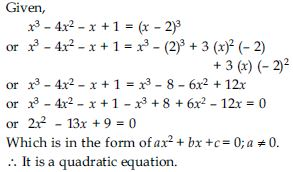##### Question 9:

Represent the following situations in the form of quadratic equations:
The area of a rectangular plot is 528 m2. The length of the plot (in metres) is one more than twice its breadth. We need to find the length and breadth of the plot.

Let breadth of rectangular plot = x m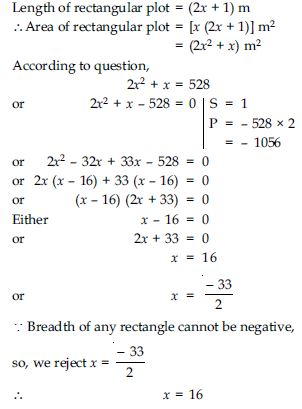Hence, breadth of rectangular plot = 16 m
Length of rectangular plot
= (2 × 16 + 1) m = 33 m
and given problem in the form of quadratic
equation is 2x2 + x - 528 = 0.

##### Question 10:

Represent the following situations in the form of quadratic equations:
The product of two consecutive positive integers is 306. We need to find the integers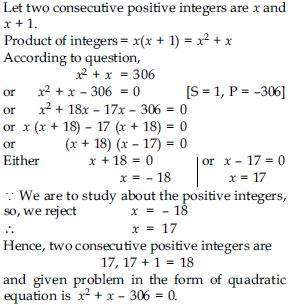##### Question 11:

Represent the following situations in the form of quadratic equations:
Rohan’s mother is 26 years older than him. The product of their ages (in years) 3 years from now will be 360. We would like to find Rohan’s present age.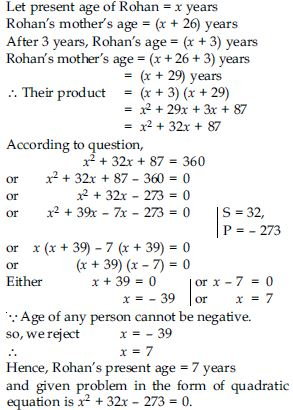##### Question 12:

Represent the following situations in the form of quadratic equations:
A train travels a distance of 480 km at a uniform speed. If the speed had been 8 km/h less, then it would have taken 3 hours more to cover the same distance. We need to find the speed of the train.

Let u km/hour be the speed of train.
Distance covered by train = 480 km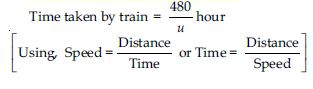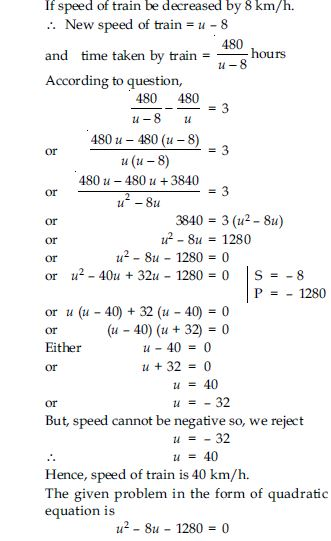##### Question 13:

Find the roots of the following quadratic equations by factorisation:
x2 - 3x - 10 = 0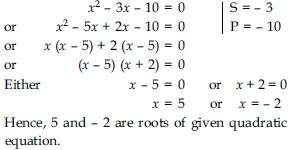##### Question 14:

Find the roots of the following quadratic equations by factorisation:
2x2 + x - 6 = 0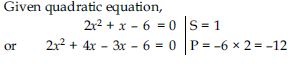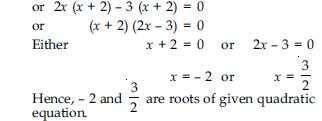##### Question 15:

Find the roots of the following quadratic equations by factorisation: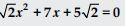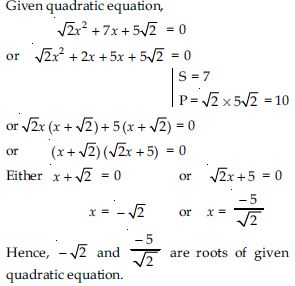##### Question 16:

Find the roots of the following quadratic equations by factorisation: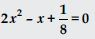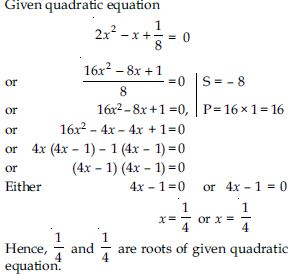##### Question 17:

Find the roots of the following quadratic equations by factorisation:
100x2 - 20x + 1 = 0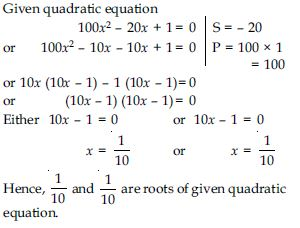##### Question 18:

Solve the problems given in Example 1. Statements of these problems are given below:
John and Jivanti together have 45 marbles. Both of them lost 5 marbles each, and the product of the number of marbles they now have is 124. We would like to find out how many marbles they had to start with.

Let the number of marbles John had be x.
Then the number of marbles Jivanti had
= 45 - x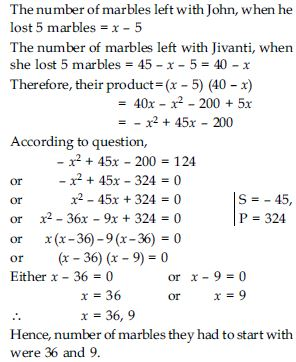##### Question 19:

Solve the problems given in Example 1. Statements of these problems are given below:
A cottage industry produces a certain number of toys in a day. The cost of production of each toy (in rupees) was found to be 55 minus the number of toys produced in a day. On a particular day, the total cost of production was ₹750. We would like to find out the number of toys produced on that day.

Let the number of toys produced on that day be x.
Therefore, the cost of production (in rupees) of each toy that day = 55 - x
So, the total cost of production (in rupees) that day = x (55 - x)
According to question,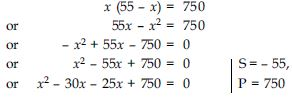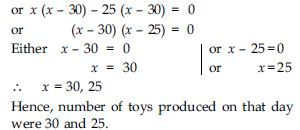##### Question 20:

Find two numbers whose sum is 27 and product is 182.

Let the first number be x.
2nd number be 27 - x.
Their product = x (27 - x) = 27x - x2
According to question,
27x - x2 = 182
or - x2 + 27x - 182 = 0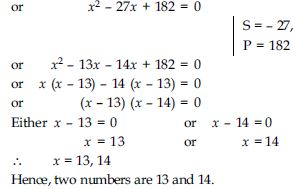##### Question 21:

Find two consecutive positive integers, sum of whose squares is 365.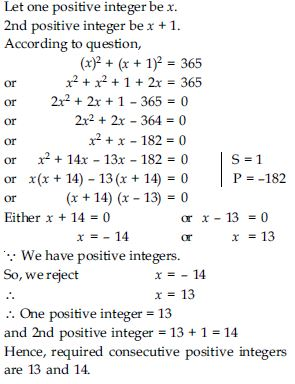##### Question 22:

The altitude of a right triangle is 7 cm less than its base. If the hypotenuse is 13 cm, find the other two sides.

Let base of right triangle be x cm.
Altitude of right triangle be (x - 7) cm.
and hypotenuse of right triangle be 13 cm.
(Given)
According to Pythagoras theorem,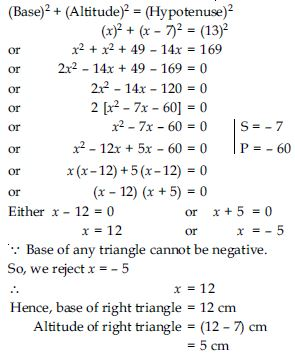##### Question 23:

A cottage industry produces a certain number of pottery articles in a day. It was observed on a particular day that the cost of production of each article (in rupees) was 3 more than twice the number of articles produced on that day. If the total cost of production on that day was ₹90, find the number of articles produced and the cost of each article.

Let, number of pottery articles produced by
industry in one day be x.
Cost of production of each article
= ₹(2x + 3)
∴ Total cost of production on a particular day
= ₹[x (2x + 3)]
= ₹(2x2 + 3x)
According to question,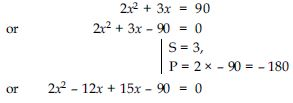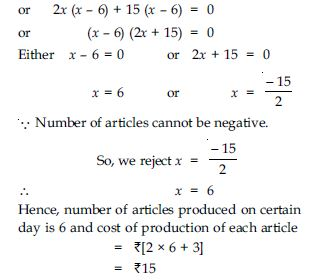##### Question 24:

Find the roots of the following quadratic equations, if they exist, by the method of completing the square:
2x2 - 7x + 3 = 0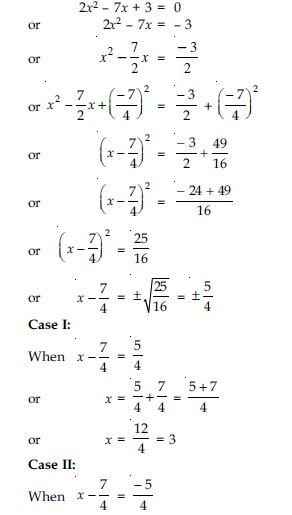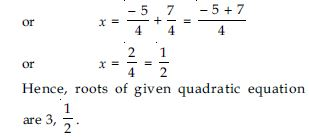##### Question 25:

Find the roots of the following quadratic equations, if they exist, by the method of completing the square:
2x2 + x - 4 = 0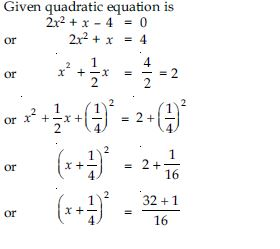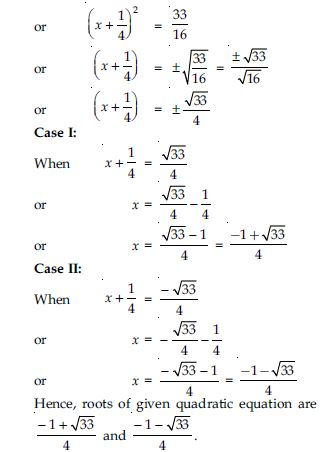##### Question 26:

Find the roots of the following quadratic equations, if they exist, by the method of completing the square: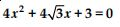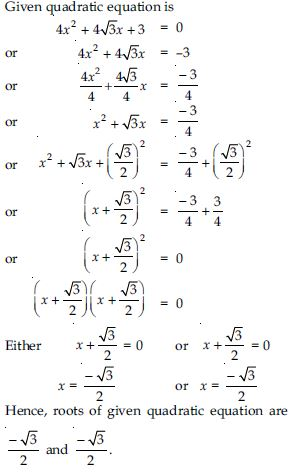##### Question 27:

Find the roots of the following quadratic equations, if they exist, by the method of completing the square:
2x2 + x + 4 = 0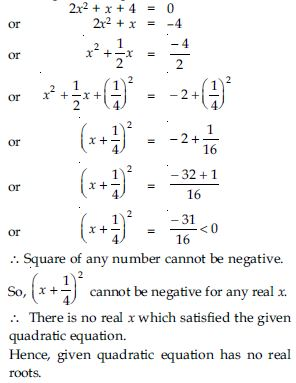##### Question 28:

Find the roots of the quadratic equations given in Q. 1 above by applying the quadratic formula.

1. 2x2 - 7x + 3 = 0
2. 2x2 + x - 4 = 0
3.4. 2x2 + x + 4 = 0

2x2 - 7x + 3 = 0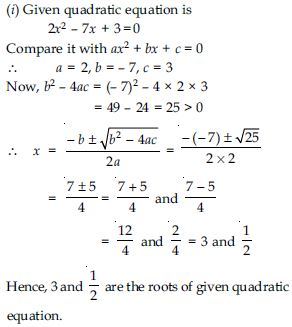(ii) Given quadratic equation is 2x2 + x - 4 = 0
Compare it with ax2 + bx + c = 0
∴ a = 2, b = 1, c = - 4
Now,
b2 - 4ac=(1)2 - 4 × 2 × (- 4)
=1 + 32 = 33 > 0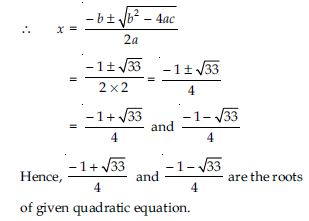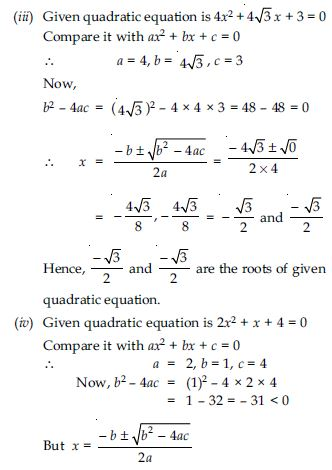Since, the square of a real number cannot be negative, therefore x will not have any real value.
Hence, there are no real roots for the given quadratic equation.

##### Question 29:

Find the roots of the following equations: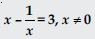Given equation is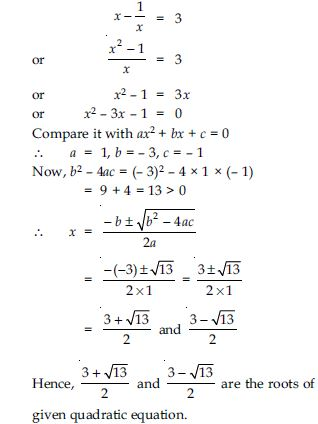##### Question 30:

Find the roots of the following equations: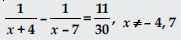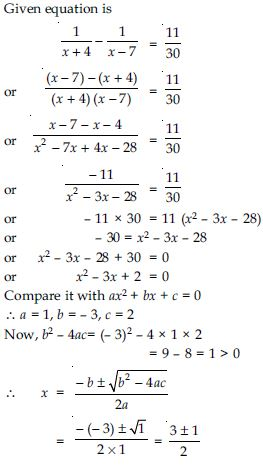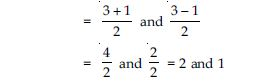Hence, 2 and 1 are the roots of given quadratic equation.

##### Question 31: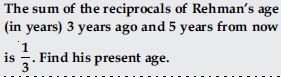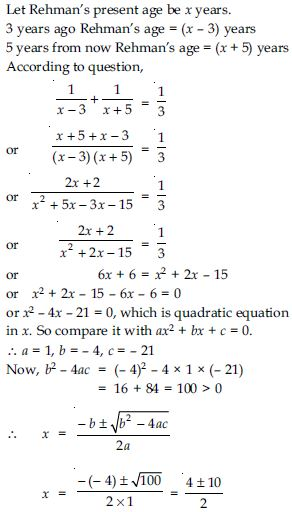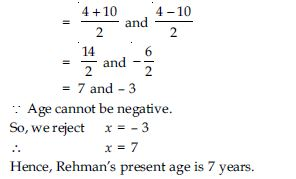##### Question 32:

In a class test, the sum of Shefali’s marks in Mathematics and English is 30. Had she got 2 marks more in Mathematics and 3 marks less in English, the product of their marks would have been 210. Find her marks in the two subjects.

Let Shefali’s marks in Mathematics be x.
∴ Shefali’s marks in English = 30 - x
According to 1st condition,
Shefali’s marks in Mathematics = x + 2
and Shefali’s marks in English = 30 - x - 3
= 27 - x
∴ The product = (x + 2) (27 - x)
= 27x - x2 + 54 - 2x
= - x2 + 25x + 54
According to 2nd condition,
- x2 + 25x + 54 = 210
or - x2 + 25x + 54 - 210 = 0
or - x2 + 25x - 156 = 0
or x2 - 25x + 156 = 0
Which is a quadratic equation in x. So, compare
it with ax2 + bx + c = 0.
∴ a = 1, b = - 25, c = 156
Now, b2 - 4ac = (- 25)2 - 4 × 1 × 156
= 625 - 624 = 1 > 0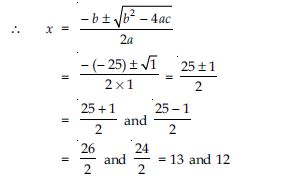Case I:
When x = 13
then Shefali’s marks in Maths = 13
Shefali’s marks in English = 30 - 13 = 17
Case II:
When x = 12
then Shefali’s marks in Maths = 12
Shefali’s marks in English = 30 - 12 =18
Hence, Shefali’s marks in two subjects are 13
and 17 or 12 and 18.

##### Question 33:

The diagonal of a rectangular field is 60 metres more than the shorter side. If the longer side is 30 metres more than the shorter side, find the sides of the field.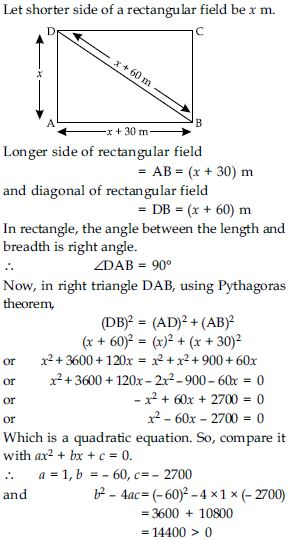∵Length of any side cannot be negative
So, we reject x = - 30
∴ x = 90
Hence, shorter side of rectangular field
= 90 m
Longer side of rectangular field
= (90 + 30) m = 120 m.

##### Question 34:

The difference of squares of two numbers is 180. The square of the smaller number is 8 times the larger number. Find the two numbers.

Let larger number = x
Smaller number = y
According to 1st condition,
x2 - y2 = 180 ...(1)
According to 2nd condition,
y2 = 8x ...(2)
From (1) and (2), we get
x2 - 8x = 180
or x2 - 8x - 180 = 0
Which is a quadratic equation. So, compare it
with ax2 + bx + c = 0.
∴ a = 1, b = - 8, c = - 180
and b2 - 4ac = (- 8)2 - 4 × 1 × (- 180)
= 64 + 720 = 784 > 0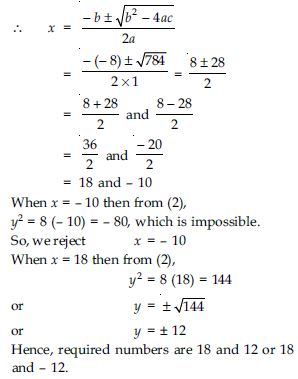##### Question 35:

A train travels 360 km at a uniform speed. If the speed had been 5 km/h more, it would have taken 1 hour less for the same journey. Find the speed of the train.

Let constant speed of the train be x km/h.
Distance covered by the train be 360 km.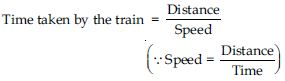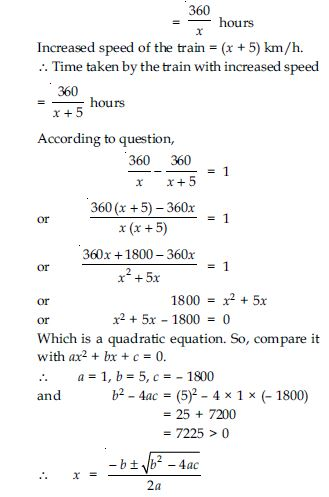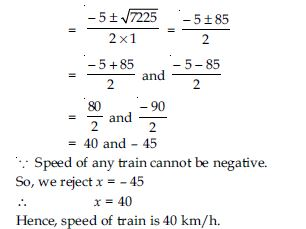##### Question 36: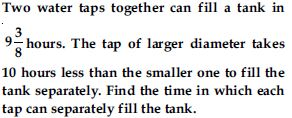Let time taken by larger tap to fill the tank be x hours.
Time taken by smaller tap to fill the tank
= (x + 10) hours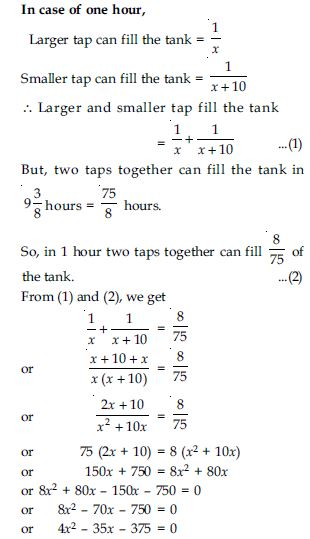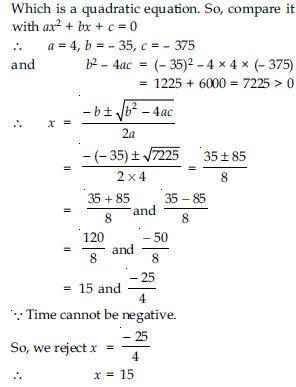Hence, larger water tap fills the tank in 15 hours and smaller water tap fills the tank in (15 + 10) hours = 25 hours.

##### Question 37:

An express train takes 1 hour less than a passenger train to travel 132 km between Mysore and Bangalore (without taking into consideration the time they stop at intermediate stations). If the average speed of the express train is 11 km/h more than that of the passenger train, find the average speed of the two trains.

Let average speed of passenger train = x km/h.
Average speed of express train = (x + 11) km/h.
Distance between Mysore and Bangalore
= 132 km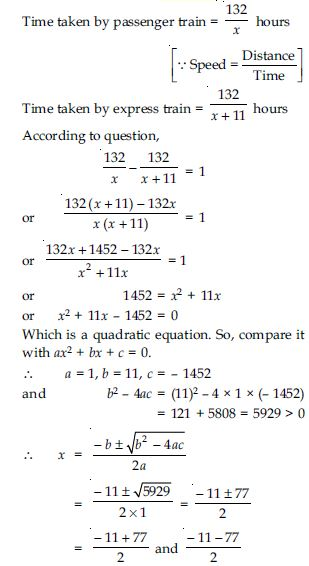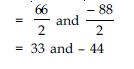∵ Speed of any train cannot be negative.
∴ x = 33
Hence, speed of passenger train = 33 km/h
and speed of express train = (33 + 11) km/h
= 44 km/h.

##### Question 38:

Sum of the areas of two squares is 468 m2. If the difference of their perimeters is 24 m, find the sides of the two squares.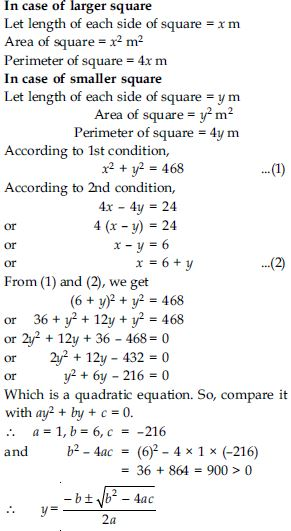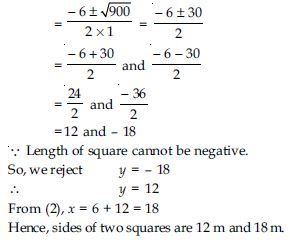##### Question 39:

Find the nature of the roots of the following quadratic equations. If the real roots exist, find them:
2x2 - 3x + 5 = 0

2x2 - 3x + 5 = 0
Compare it with ax2 + bx + c = 0
∴ a = 2, b = - 3, c = 5
D = b2 - 4ac
= (- 3)2 - 4 × 2 × 5 = 9 - 40 = - 31 $<$ 0
Hence, the quadratic equation has no real roots.

##### Question 40:

Find the nature of the roots of the following quadratic equations. If the real roots exist, find them: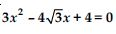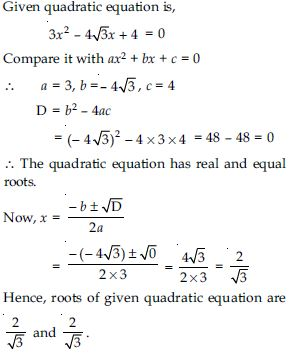##### Question 41:

Find the nature of the roots of the following quadratic equations. If the real roots exist, find them:
2x2 - 6x + 3 = 0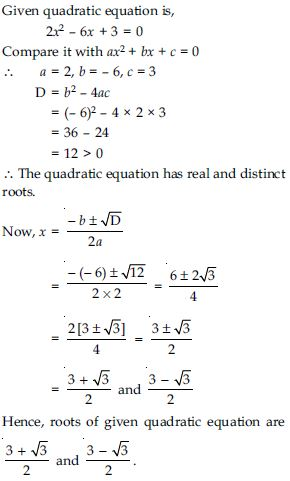##### Question 42:

Find the values of k for each of the following quadratic equations, so that they have two equal roots.
2x2 + kx + 3 = 0

2x2 + kx + 3 = 0
Compare it with ax2 + bx + c = 0
∴ a = 2, b = k, c = 3
∵ Roots of the given quadratic equation are equal.
∴ D = 0
b2 - 4ac = 0
or (k)2 - 4 × 2 × 3 = 0
or k2 - 24 = 0
or k2 = 24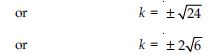##### Question 43:

Find the values of k for each of the following quadratic equations, so that they have two equal roots.
kx (x - 2) + 6 = 0

kx (x - 2) + 6 = 0
or kx2 - 2kx + 6 = 0
Compare it with ax2 + bx + c = 0
∴ a = k, b = - 2k, c = 6
∵Roots of the given quadratic equation are
equal.
∴ D = 0
b2 - 4ac = 0
or (- 2k)2 - 4 × k × 6 = 0
or 4k2 - 24k = 0
or 4k [k - 6] = 0
Either 4k = 0 or k - 6 = 0
k = 0 or k = 6
∴ k = 0, 6.

##### Question 44:

Is it possible to design a rectangular mango grove whose length is twice its breadth, and the area is 800 m2? If so, find its length and breadth.

Let breadth of rectangular grove be x m.
and length of rectangular grove = 2x m
Area of rectangular grove = Length × Breadth
= [x × 2x] m2 = 2x2 m2
According to question,
2x2 = 800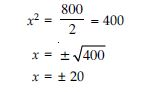∵ A side of rectangle cannot be negative.
So, we reject x = - 20
∴ x = 20
∴Breadth of rectangular grove = 20 m
and length of rectangular grove
= (2 × 20) m = 40 m.

##### Question 45:

Is the following situation possible? If so, determine their present ages. The sum of the ages of two friends is 20 years. Four years ago, the product of their ages in years was 48.

Let age of one friend be x years.
and the age of 2nd friend = (20 - x) years
Four years ago,
Age of 1st friend = (x - 4) years
Age of 2nd friend = (20 - x - 4) years
= (16 - x) years
∴ Their product = (x - 4) (16 - x)
= 16x - x2 - 64 + 4x
= - x2 + 20x - 64
According to question,
- x2 + 20x - 64 = 48
or - x2 + 20x - 64 - 48 = 0
or - x2 + 20x - 112 = 0
or x2 - 20x + 112 = 0 ...(1)
Compare it with ax2 + bx + c = 0
∴ a = 1, b = - 20, c = 112
D = b2 - 4ac
= (- 20)2 - 4 × 1 × 112
= 400 - 448 = - 48 $<$ 0
∴ Roots are not real.
Then no real value of x satisfies the quadratic equation (1).
Hence, given situation is not possible.

##### Question 46:

Is it possible to design a rectangular park of perimeter 80 m and area 400 m2? If so, find its length and breadth.